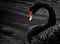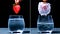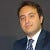# How to Interpret the Logistic Regression model — with Python

Logistic regression model is one of the efficient and pervasive classification methods for the data science.

Many business problems require automating decisions. For example, what is the churn likelihood for a given customer? What is the likelihood of a click on an ad for a given customer? and so many alike. These are categorised as classification problems which itself is a part of larger topic called supervised learning. Most of the classification problems have an outcome which takes only two different values. These sort of classification problems are known as binary classification. Some examples of binary outcomes are phishing/not-phishing, click/don’t…

# Discriminant Analysis for Data Science: Python

Linear discriminant analysis is one of the earliest classification algorithms in machine learning.

Many business problems require automating decisions such as what is the churn likelihood for a given customer? What is the likelihood of a click on an ad for a given customer? and so many alike. These are categorised as classification problems which itself is a part of larger topic called supervised learning. Most of the classification problems have an outcome which takes only two different values. These classification problems are known as binary classification. Some examples of binary outcomes are phishing/not-phishing, click/don’t click, churn/don’t churn. …

# Naive Bayes for Data Science — With Python

Naive Bayesian method is one of the efficient algorithms for classification purpose.

Many business problems require automating decisions. For example, what is the churn likelihood for a given customer? What is the likelihood of a click on an ad for a given customer? and so many alike. These are categorised as classification problems which itself is a part of larger topic called supervised learning. Most of the classification problems have an outcome which takes only two different values. These classification problems are known as binary classification. Some examples of binary outcomes are phishing/not-phishing, click/don’t click, churn/don’t churn. …

# Diagnose your Linear Regression Model — With Python

Regression diagnostics is a critical step in reaching a meaningful regression model.

One of the main interests of statisticians and data scientists is examining the association of one single variable, called target or response, with another set of variables, called predictors, for prediction or profiling purposes. The common steps involved in reaching this goal include but not limited to:

• Pre-processing data
• Examining the data
• Model selection
• Training the model
• Model diagnostic

Most of the articles in the machine learning domain cover the first four steps but less emphasise the last step which might be the most important step in decision…

# Linear Regression for Data Science — With Python

The linear regression is the cornerstone of the data-driven decision-making process in data science.

Both data scientists and statisticians share the task of predicting one output variable, called target variable, based on one or set of variables, called predictors. Nowhere is the nexus between statistics and data science stronger than in the realm of prediction. Linear regression is proved to be a powerful tool in extracting the association between target output and predictors. Using the linear regression, we aim at not only quantifying the intensity of the relation but also on the nature of this relation. In other words, by…

# Significance Tests: t-Test, F-Statistic, ANOVA and More — with Python

There are numerous types of significance tests to evaluate the experiment result.

The significance tests are used to not let humans get fooled by the randomness. Humans have a tendency to interpret the world around according to some patterns which might not exist at all and all there is just a complete randomness. In fact, we underestimate the share of randomness in our lives. However, the randomness plays a critical role in our daily lives which should be given credit when the credit is due. This illusion is famous as it deserves an exclusive name for it: Black Swan phenomenan!A Black Swan

# Significance Testing for Data Science — with Python Implementation

Confirming and rejecting a hypothesis is a cornerstone of the practice of statistics.

Data scientists often need to conduct a continual statistical experiment in the context of for example user interface and product marketing. In classic statistics, the statisticians mostly limit their attention on the inference, as a complex procedure on drawing a conclusion for a population based on the limited number of samples.Hypothesis Testing

The aim of inference is to generalise the result of limited size of sampling data to a larger set of population data.

# What is the Sampling Distribution of a Statistic?

Sampling distribution of statistic is the main step in statistical inference.

In classic statistics, the statisticians mostly limit their attention on the inference, as a complex procedure on drawing a conclusion for a population based on the limited number of samples. For example, we want to know what the gender pay gap is in Australia. Strictly speaking, what is the Australians’ average pay gap? In order to answer similar questions, we collect data called sampling from larger data set known as population which is usually theoretical or imaginary in the sense that we do not access to the whole data…

# What is the Sampling Bias?

Drawing a valid conclusion depends heavily on how you collect data.

In many statistical analysis and data-driven decisions, we need to draw an actionable conclusion, supported by the data collected already or needed to be collected. However, the quality of conclusion derived from the data heavily depends on the quality of the data you collected. During data collection, you sample data usually from a larger data set. In most of cases, you do not have luxury to collect the data from all cases and hence measure the intended metrics truely. However, you can infer some characteristics of the larger dataset…

# The First Chapter of Data Analysis — Part II

Explore how the data is distributed overall.

In the first part, we found the basis for the exploratory data analysis (EDA) with focus on the statistics summary. In this part, we will exclusively look at the data distribution and investigate different approaches in representing the data.

Using the statistics summary, we gain an insight on the abstract of the data in the form of numerical values such as location and variability. This is particularly important in univariate analysis where only one single variable is of interest. …## Vahid Naghshin

Technology enthusiast, Futuristic, Telecommunications, Machine learning and AI savvy, work at Dolby Inc.